Math Quiz For Everybody
Question 1 of 10
What is the remainder when we divide 30 by 4?
Question 2 of 10
What is the distance around a shape called?
Question 3 of 10
What is 3/4 in decimal form?
Question 4 of 10
What is the perimeter of a circle also called?
Question 5 of 10
What does the 8 mean in the number 582?
Question 6 of 10
What are the factors in this problem: 2 x 8 = 16?
Question 7 of 10
What is 3291 rounded to the nearest 1000?
Question 8 of 10
A mixed number contains what two elements?
Question 9 of 10
In the fraction 3/4, which number is the denominator?
Question 10 of 10
What does the symbol ">" mean in math?General Knowledge For Trivia L
Share with your friends if you enjoyed the quiz!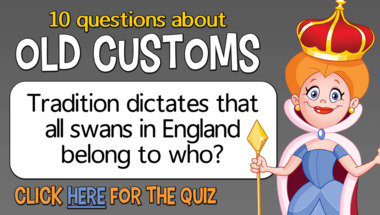Trivia Quiz About The Year 197
10 Hard Questions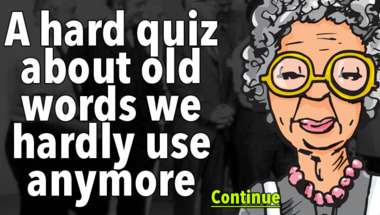Trivia quiz for smart people
How many correct will you get?1957 History Quiz
How many correct will you get?Trivia Quiz About The Year 197
Only 10% will get these questions right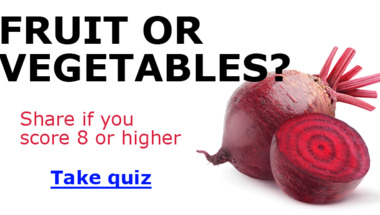General Trivia Quiz
How many correct will you get?1960s Quiz
How many of them do you remember?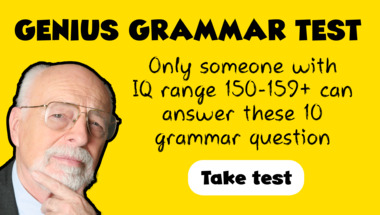Quiz : Which of these songs is
How many of them will you answer correctly?Quiz : Help us complete these
10 songs to guess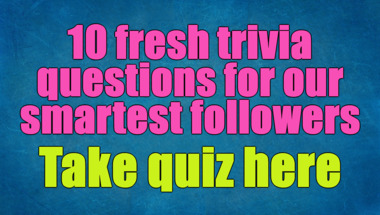Spelling Test
10 words that will put your skills to the test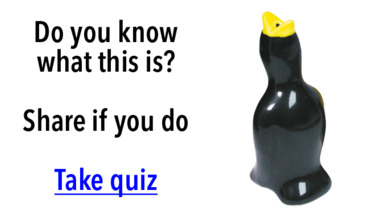General Trivia Quiz
10 questions to test your knowledge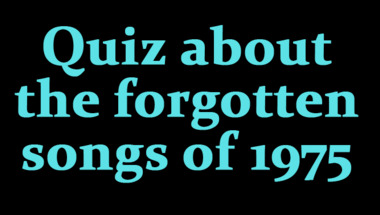Trivia quiz for smart people
How many correct will you get?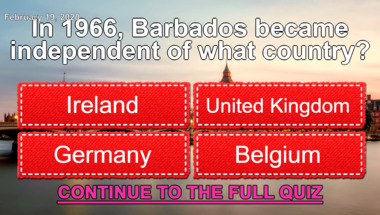1950s Song Quiz
How many correct will you get?Trivia quiz for smart people
Scoring a clean 10 will never happen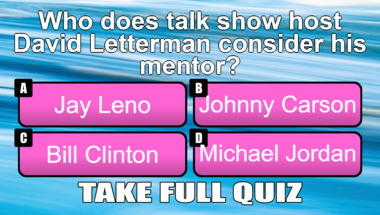10 Completely Impossible Trivi
Not Even a Trivia Expert Will Get Close To 10 Correct Answers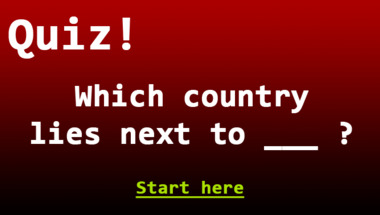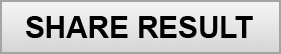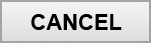Copyright 2019 - Wicked Media ApS
Contact | Privacy Policy